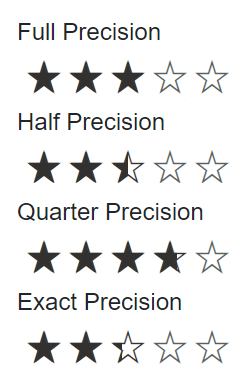# Precision Modes in Blazor Rating Component

12 Jan 20231 minute to read

You can use the Precision property of the Blazor Rating component to provide ratings with varying levels of precision.

The precision types of Rating are as follows:

• Full: The rating is increased in whole number increments. For example, if the current rating is 2, the next possible ratings are 3, 4, and so on.
• Half: The rating is increased in increments of 0.5 (half). For example, if the current rating is 2.5, the next possible ratings are 3, 3.5, 4, and so on.
• Quarter: The rating is increased in increments of 0.25 (quarter). For example, if the current rating is 3.75, the next possible ratings are 4, 4.25, 4.5, and so on.
• Exact: The rating is increased in increments of 0.1. For example, if the current rating is 3.9, the next possible ratings are 4, 4.1, 4.2, and so on.
``````@using Syncfusion.Blazor.Inputs

<label>Full Precision</label><br/>
<SfRating Value="3" Precision="PrecisionType.Full"></SfRating><br/>

<label>Half Precision</label><br/>
<SfRating Value="2.5" Precision="PrecisionType.Half"></SfRating><br/>

<label>Quarter Precision</label><br/>
<SfRating Value="3.75" Precision="PrecisionType.Quarter"></SfRating><br/>

<label>Exact Precision</label><br/>
<SfRating Value="2.3" Precision="PrecisionType.Exact"></SfRating><br/>``````